# Nuclear Reactions Transmutations Fission and Fusion Nuclear reactions

• Slides: 39Nuclear Reactions, Transmutations, Fission and Fusion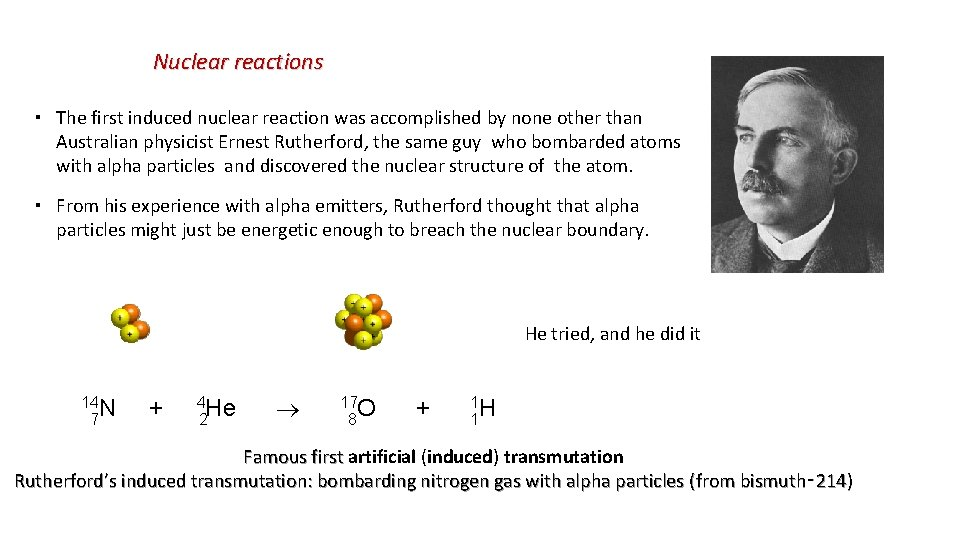Nuclear reactions ▪ The first induced nuclear reaction was accomplished by none other than Australian physicist Ernest Rutherford, the same guy who bombarded atoms with alpha particles and discovered the nuclear structure of the atom. ▪ From his experience with alpha emitters, Rutherford thought that alpha particles might just be energetic enough to breach the nuclear boundary. He tried, and he did it 14 N + 4 He 17 O + 1 H 7 2 8 1 Famous first artificial (induced) transmutation first Rutherford’s induced transmutation: bombarding nitrogen gas with alpha particles (from bismuth‑ 214)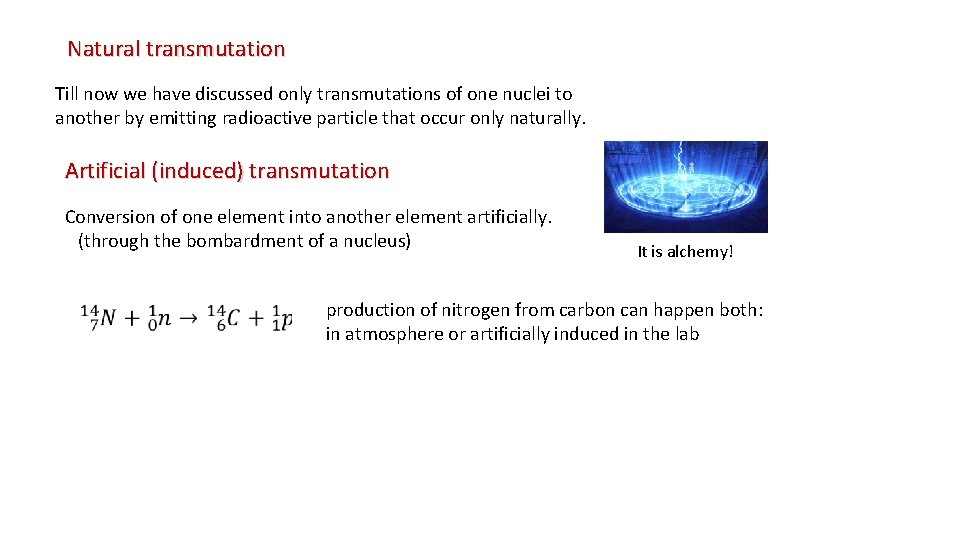Natural transmutation Till now we have discussed only transmutations of one nuclei to another by emitting radioactive particle that occur only naturally. Artificial (induced) transmutation Conversion of one element into another element artificially. (through the bombardment of a nucleus) It is alchemy! production of nitrogen from carbon can happen both: in atmosphere or artificially induced in the lab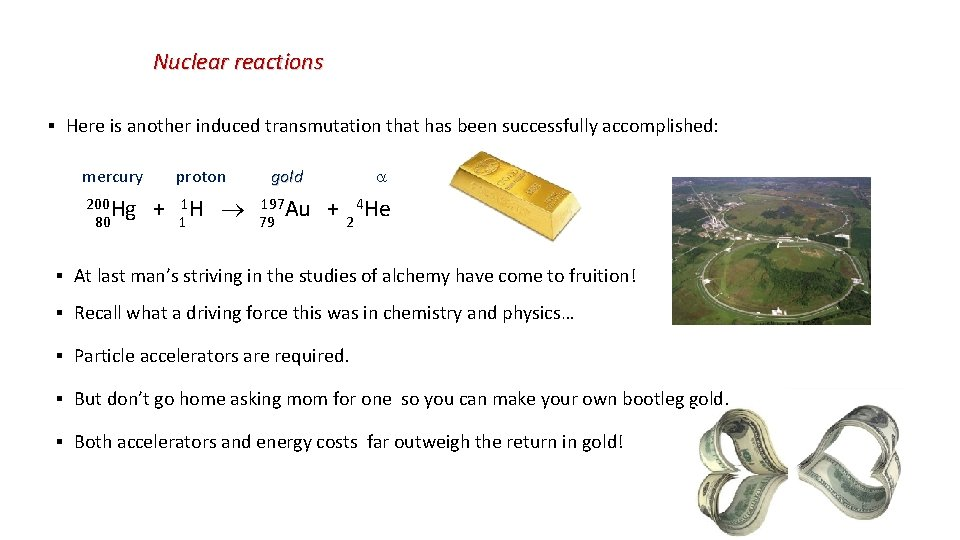Nuclear reactions ▪ Here is another induced transmutation that has been successfully accomplished: mercury proton gold 200 Hg + 1 H 197 Au + 4 He 80 1 79 2 ▪ At last man’s striving in the studies of alchemy have come to fruition! ▪ Recall what a driving force this was in chemistry and physics… ▪ Particle accelerators are required. ▪ But don’t go home asking mom for one so you can make your own bootleg gold. ▪ Both accelerators and energy costs far outweigh the return in gold!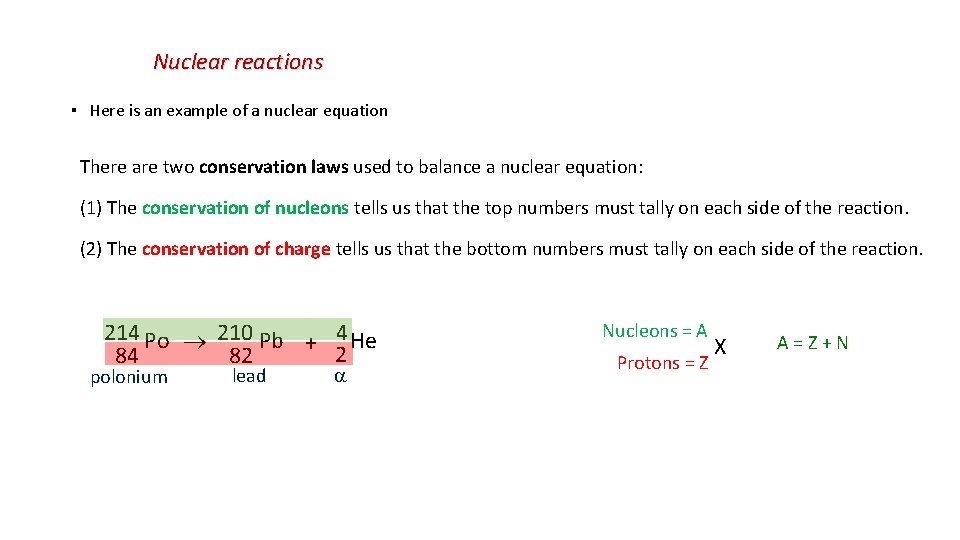Nuclear reactions ▪ Here is an example of a nuclear equation There are two conservation laws used to balance a nuclear equation: (1) The conservation of nucleons tells us that the top numbers must tally on each side of the reaction. (2) The conservation of charge tells us that the bottom numbers must tally on each side of the reaction. 214 Po 210 Pb + 4 He 2 84 82 polonium lead Nucleons = A Protons = Z X A = Z + N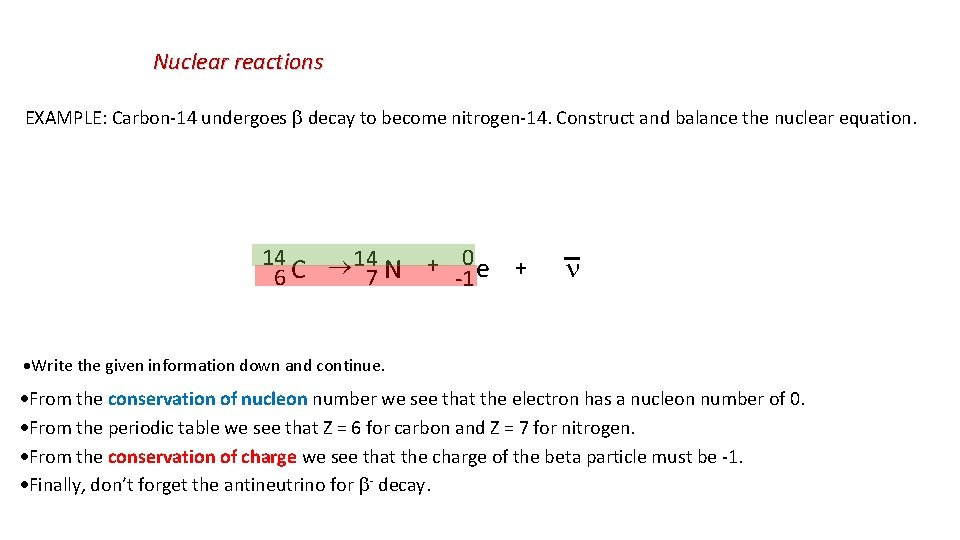Nuclear reactions EXAMPLE: Carbon-14 undergoes decay to become nitrogen-14. Construct and balance the nuclear equation. 14 N C 6 7 + 0 e -1 + Write the given information down and continue. From the conservation of nucleon number we see that the electron has a nucleon number of 0. From the periodic table we see that Z = 6 for carbon and Z = 7 for nitrogen. From the conservation of charge we see that the charge of the beta particle must be -1. Finally, don’t forget the antineutrino for - decay.Nuclear reactions Just know this!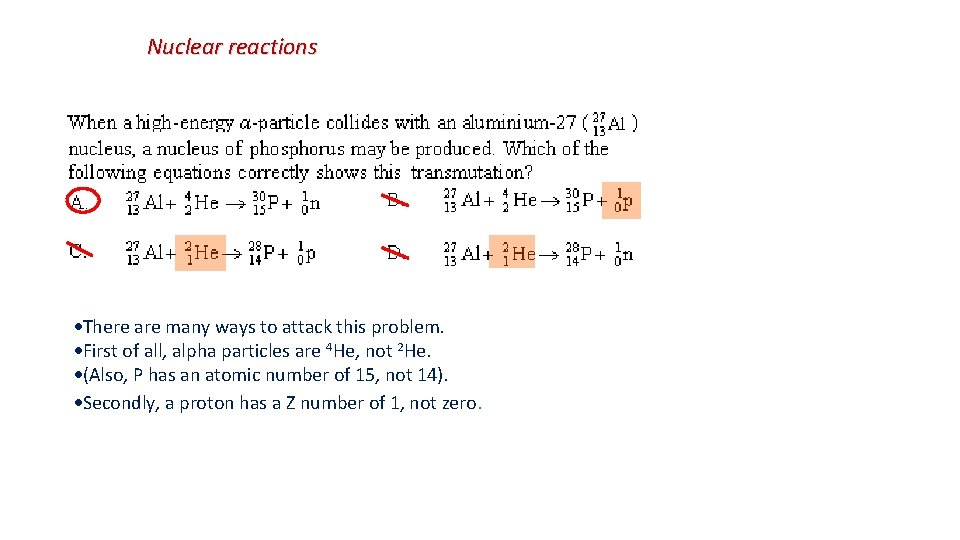Nuclear reactions There are many ways to attack this problem. First of all, alpha particles are 4 He, not 2 He. (Also, P has an atomic number of 15, not 14). Secondly, a proton has a Z number of 1, not zero.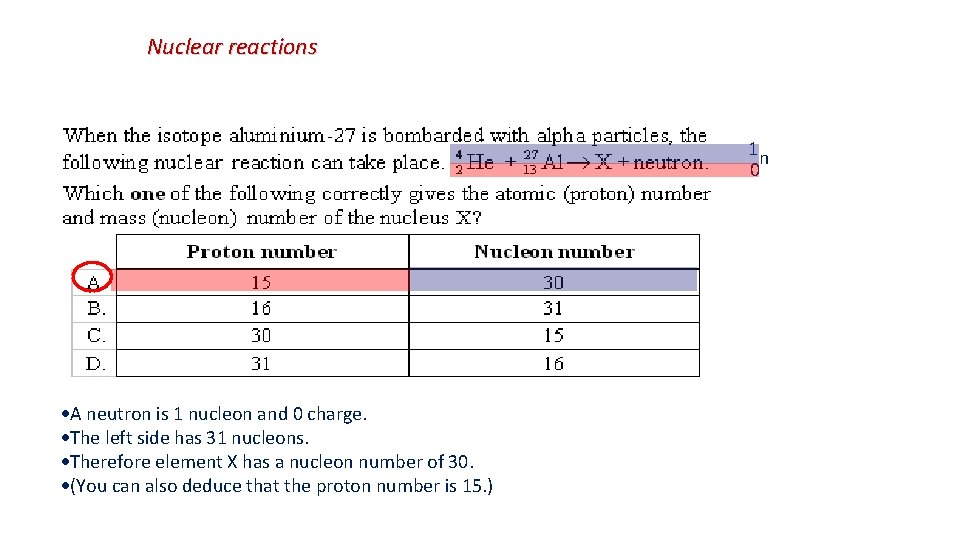Nuclear reactions 1 n 0 A neutron is 1 nucleon and 0 charge. The left side has 31 nucleons. Therefore element X has a nucleon number of 30. (You can also deduce that the proton number is 15. )Nuclear reactions 0 + -1 e + The nucleon number is already balanced. The charge on the right is high. These two numbers are characteristic of the electron. The process is beta-minus decay. Don’t forget the antineutrino that goes with beta-minus.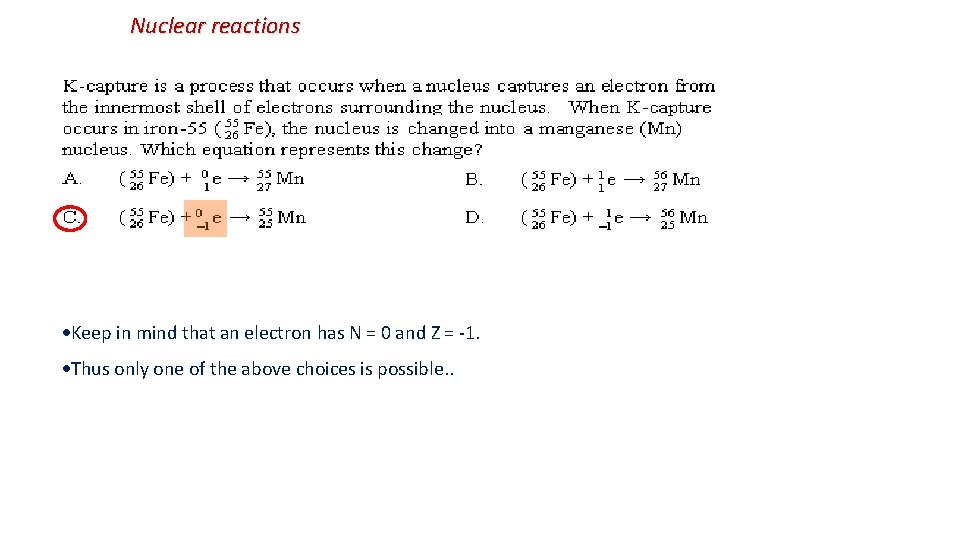Nuclear reactions Keep in mind that an electron has N = 0 and Z = -1. Thus only one of the above choices is possible. .The unified atomic mass unit (amu abbreviated as u) ▪ In the world of nuclear reactions we have to keep very precise track of the mass of the nuclei if we are to determine the energy of a reaction. 1 u = 1/12 of the mass of one atom of carbon-12 (6 p+6 n+6 e) ▪ 1 u = 1. 66053886 x 10 -27 kgEinstein’s mass-energy equivalence relationship ▪ To understand nuclear reactions we have to use Einstein’s mass-energy equivalence relationship: ▪ In 1905, while developing his special theory of relativity, Einstein made the startling suggestion that energy and mass are equivalent E = mc 2 where c is the speed of light in vacuum. This equation is probably the most famous of all. What E = mc 2 means is that mass and energy are interchangeable. PROBLEM: How many students does it take to power a lightbulb? Wanna know what you’re worth, energy-wise? It’s pretty easy to figure out! Take an average student, of about 70 kg. So if we turn this whole student from mass into energy, we get: E = 6. 3 x 1018 J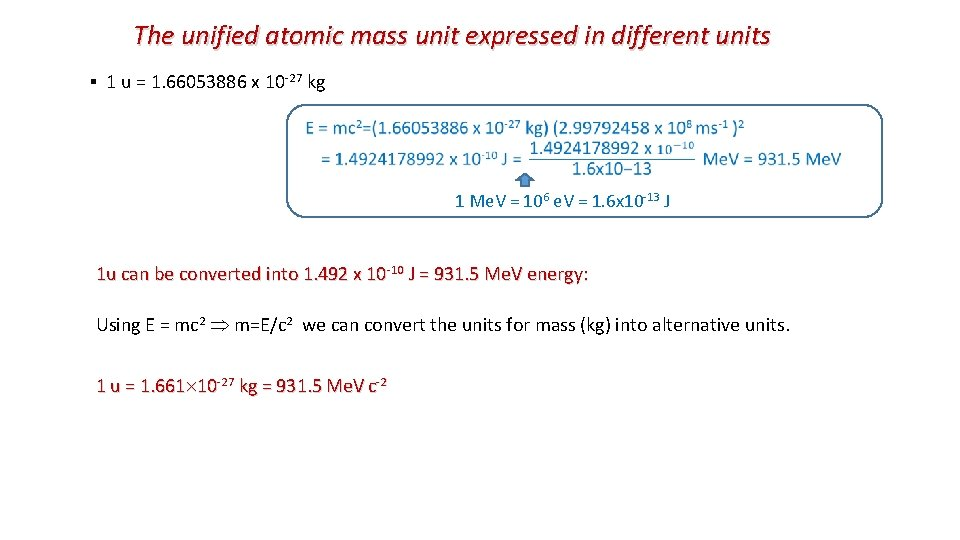The unified atomic mass unit expressed in different units ▪ 1 u = 1. 66053886 x 10 -27 kg 1 Me. V = 106 e. V = 1. 6 x 10 -13 J 1 u can be converted into 1. 492 x 10 -10 J = 931. 5 Me. V energy: Using E = mc 2 m=E/c 2 we can convert the units for mass (kg) into alternative units. 1 u = 1. 661 10 -27 kg = 931. 5 Me. V c-2Two situations when we use Einstein’s mass-energy equivalence relationship in nuclear physics: 1. Mass defect and nuclear binding energy ▪ When nucleons bind together to form nucleus the mass of the nucleus is found to be less than the sum of the masses of the constituent protons and neutrons. ▪ On the other hand the sum of the masses of the constituent free protons and neutrons is found to be more than the mass of original nucleus. ▪ The only possible conclusion: mass is converted into energy and energy is converted into mass The "mass defect" is therefore mass that transforms to energy according to Einstein's equation and is released in forming the nucleus from its component particles. ▪ Binding energy (BE) - is the energy required to separate the nucleus into it individual nucleons Binding energy (BE) / the energy that is released in assembling a nucleus from its individual nucleons. (heat, light, higher energy states of the nucleus …).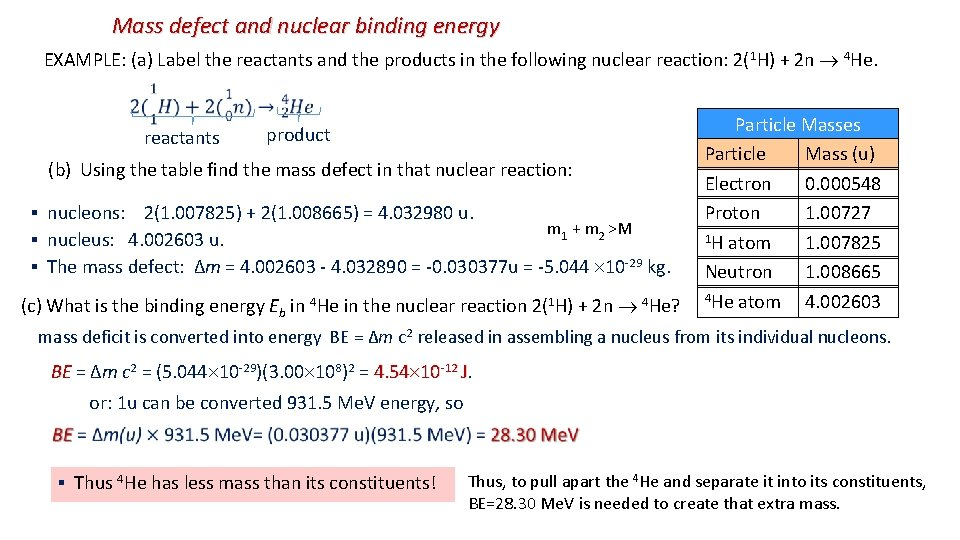Mass defect and nuclear binding energy EXAMPLE: (a) Label the reactants and the products in the following nuclear reaction: 2( 1 H) + 2 n 4 He. reactants product (b) Using the table find the mass defect in that nuclear reaction: ▪ nucleons: 2(1. 007825) + 2(1. 008665) = 4. 032980 u. m 1 + m 2 >M ▪ nucleus: 4. 002603 u. ▪ The mass defect: ∆m = 4. 002603 - 4. 032890 = -0. 030377 u = -5. 044 10 -29 kg. (c) What is the binding energy Eb in 4 He in the nuclear reaction 2(1 H) + 2 n 4 He? Particle Masses Particle Mass (u) Electron 0. 000548 Proton 1. 00727 1 H atom 1. 007825 Neutron 1. 008665 4 He atom 4. 002603 mass deficit is converted into energy BE = ∆m c 2 released in assembling a nucleus from its individual nucleons. 2 = (5. 044 10 -29)(3. 00 108)2 = 4. 54 10 -12 J. BE = ∆m c BE or: 1 u can be converted 931. 5 Me. V energy, so ▪ Thus 4 He has less mass than its constituents! Thus, to pull apart the 4 He and separate it into its constituents, BE=28. 30 Me. V is needed to create that extra mass.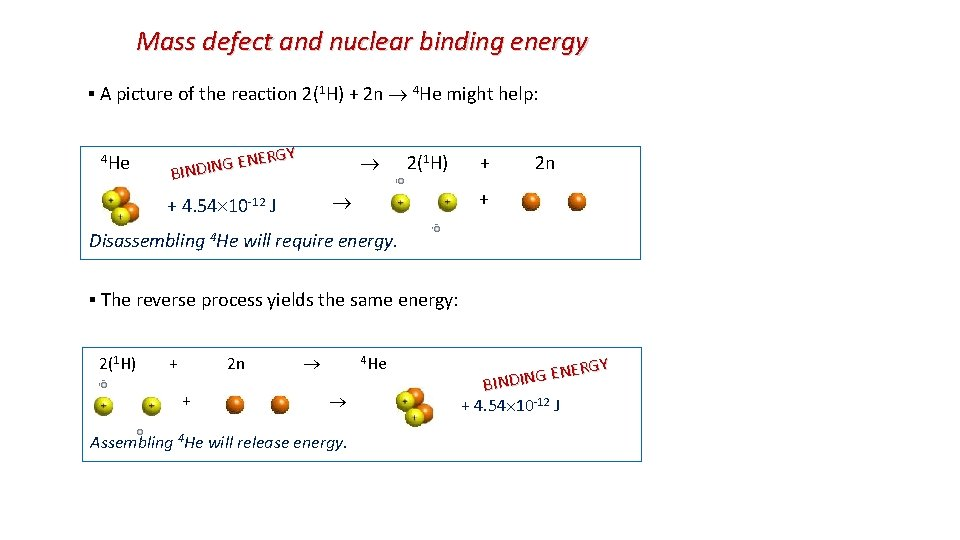Mass defect and nuclear binding energy ▪ A picture of the reaction 2(1 H) + 2 n 4 He might help: 1 H) + 2 n RGY E N E 2( G N I D BIN 4 He + 4. 54 10 -12 J + Disassembling 4 He will require energy. ▪ The reverse process yields the same energy: 2(1 H) + 2 n 4 He + Assembling 4 He will release energy. RGY E N E G N BINDI + 4. 54 10 -12 J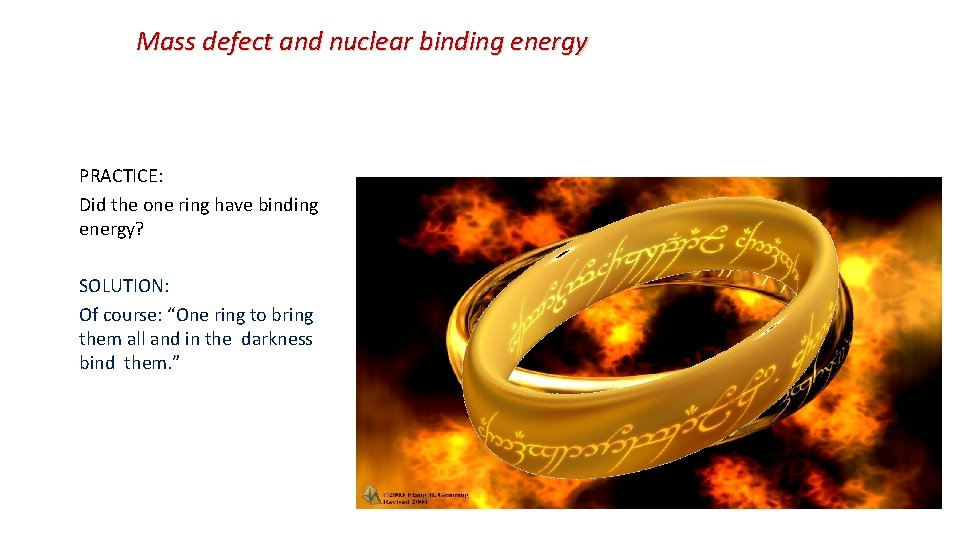Mass defect and nuclear binding energy PRACTICE: Did the one ring have binding energy? SOLUTION: Of course: “One ring to bring them all and in the darkness bind them. ”Mass defect in nuclear decay: A → C + D is positive ▪ M > m 1 + m 2 ▪ What happened to that mass difference? ▪ Energy corresponding to that mass difference is released as kinetic energy of the products Mass of aluminum-29 nucleus = 28. 97330 u Mass of silicon-29 nucleus + b-particle + antineutrino = 28. 96880 u + 0. 000549 u + 0 = 28. 969349 u ▪ Mass difference = 28. 97330 u – 28. 969349 u = 0. 003951 u BE= 3. 68 Me. V ▪ Again this becomes the kinetic energy of the decay products.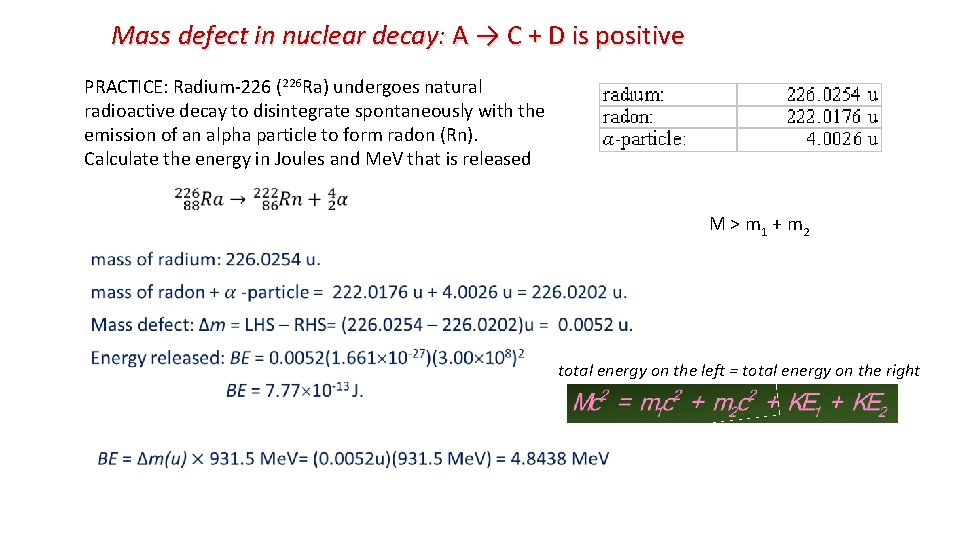Mass defect in nuclear decay: A → C + D is positive PRACTICE: Radium-226 (226 Ra) undergoes natural radioactive decay to disintegrate spontaneously with the emission of an alpha particle to form radon (Rn). Calculate the energy in Joules and Me. V that is released M > m 1 + m 2 total energy on the left = total energy on the right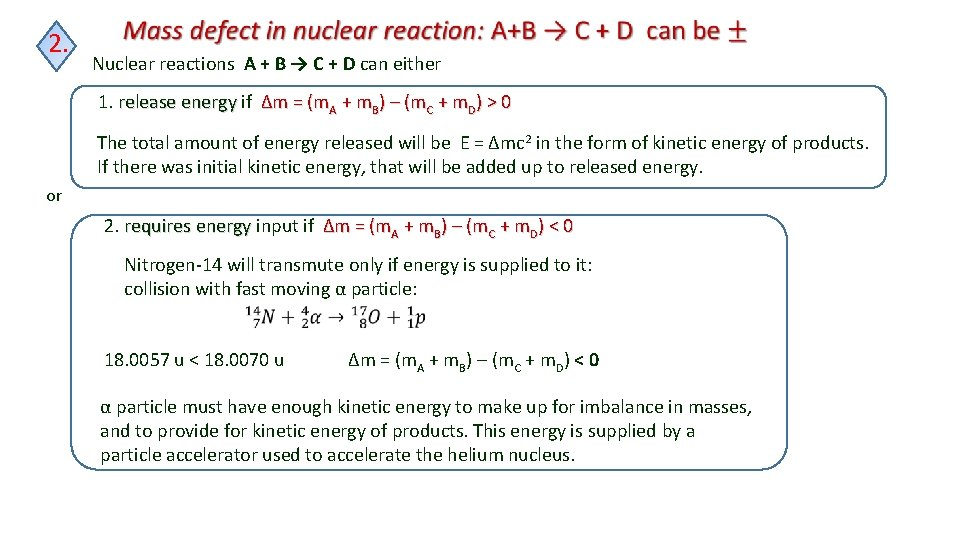2. Nuclear reactions A + B → C + D can either 1. release energy if release energy Δm = (m. A + m. B) – (m. C + m. D) > 0 The total amount of energy released will be E = Δmc 2 in the form of kinetic energy of products. If there was initial kinetic energy, that will be added up to released energy. or 2. requires energy input if Δm = (m. A + m. B) – (m. C + m. D) < 0 energy Nitrogen-14 will transmute only if energy is supplied to it: collision with fast moving α particle: 18. 0057 u < 18. 0070 u Δm = (m. A + m. B) – (m. C + m. D) < 0 α particle must have enough kinetic energy to make up for imbalance in masses, and to provide for kinetic energy of products. This energy is supplied by a particle accelerator used to accelerate the helium nucleus.Binding energy per nucleon ▪ The binding energy per nucleon BE / A of a nucleus is the binding energy BE divided by the number of nucleons A/mass number EXAMPLE: What is the binding energy per nucleon of 4 He? ▪ 4 He has a binding energy of BE = 4. 54 10 -12 J. ▪ 4 He has four nucleons (2 p and 2 n → A = 4). ▪ Thus BE / A = 1. 14 10 -12 J / nucleon. for pure Fe it is 8. 7 Me. V/nucleon ▪ Rather than looking at the total binding energy of nuclei, we often look at the binding energy per nucleon (graph). ▪ This number tells us about how difficult it is to remove each nucleon from the nucleus. ▪ The bigger the binding energy per nucleon, the more stable the nucleus. ▪ The bigger the binding energy per nucleon, the less likely a nucleus will be to want to lose one of its nucleons. ▪ Thus it is more stable, by definition.If a nucleus has a large binding energy then it will require a lot of work to pull it apart – we say it is stable. The binding energy curve Graph of binding energy per nucleon curve BE varies with mass number; BE increase as the mass (nucleon) number increases up to Fe. Fe is most stable. After that it slightly decreases. In most cases it is about 8 Me. VPRACTICE: Which is the most stable element? ▪ The bigger the binding energy per nucleon the more stable an element is. ▪ Iron-56 is the most stable element. PRACTICE: What is the binding energy of uranium-238? ▪ Note that the binding energy per nucleon of 238 U is about 7. 6 Me. V. ▪ But since 238 U has 238 nucleons, the binding energy is (238)(7. 6 Me. V) = 1800 Me. V.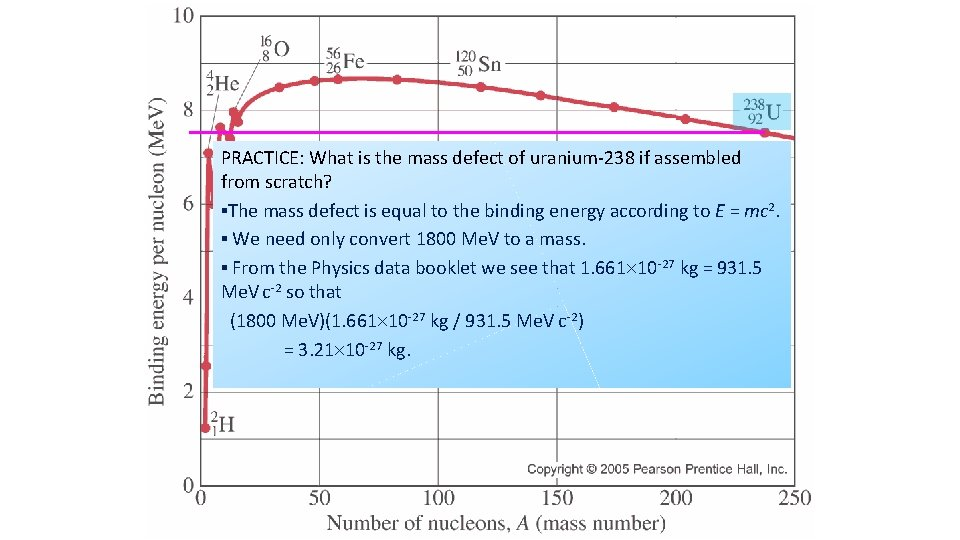PRACTICE: What is the mass defect of uranium-238 if assembled from scratch? ▪The mass defect is equal to the binding energy according to E = mc 2. ▪ We need only convert 1800 Me. V to a mass. ▪ From the Physics data booklet we see that 1. 661 10 -27 kg = 931. 5 Me. V c-2 so that (1800 Me. V)(1. 661 10 -27 kg / 931. 5 Me. V c-2) = 3. 21 10 -27 kg.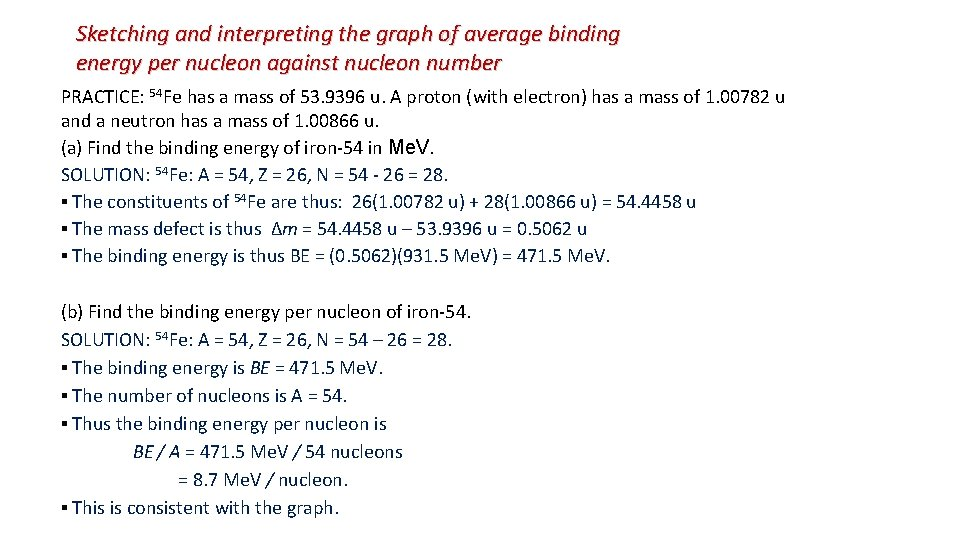Sketching and interpreting the graph of average binding energy per nucleon against nucleon number PRACTICE: 54 Fe has a mass of 53. 9396 u. A proton (with electron) has a mass of 1. 00782 u and a neutron has a mass of 1. 00866 u. (a) Find the binding energy of iron-54 in Me. V. SOLUTION: 54 Fe: A = 54, Z = 26, N = 54 - 26 = 28. ▪ The constituents of 54 Fe are thus: 26(1. 00782 u) + 28(1. 00866 u) = 54. 4458 u ▪ The mass defect is thus ∆m = 54. 4458 u – 53. 9396 u = 0. 5062 u ▪ The binding energy is thus BE = (0. 5062)(931. 5 Me. V) = 471. 5 Me. V. (b) Find the binding energy per nucleon of iron-54. SOLUTION: 54 Fe: A = 54, Z = 26, N = 54 – 26 = 28. ▪ The binding energy is BE = 471. 5 Me. V. ▪ The number of nucleons is A = 54. ▪ Thus the binding energy per nucleon is BE / A = 471. 5 Me. V / 54 nucleons = 8. 7 Me. V / nucleon. ▪ This is consistent with the graph.Nuclear fission ▪ Nuclear fission is the splitting of a large nucleus (A > 200) fission into two smaller (daughter) nuclei. ▪ Total BE would increase which means that the daughters are more stable than parent. ▪ The excess energy is released by the reaction. ▪ Spontaneous fission is very rare. Uranium is the largest nucleus found on Earth. Its isotopes will sometimes fission naturally. But half-life for U-235 is 7. 04 x 108 years ▪ Bombarding the nucleus with neutrons can trigger a fission reaction. ▪ For example 235 U + 1 n (236 U*) 140 Xe + 94 Sr + 2(1 n) 92 0 92 54 38 0 + energy 235 U is hit by a neutron, and capturing it, becomes excited and unstable: It quickly splits into two smaller daughter nuclei, and two neutrons. 94 Sr 140 XeNuclear fission The strong forces that hold the nucleus together only act over a very short distance. When a uranium nucleus absorbs a neutron it knocks the nucleus out of shape. If the nucleus deforms enough, the electrostatic repulsion between the protons in each half becomes greater than the strong force. It then splits in two. The nuclei splits randomly In the diagram, the fission fragments are shown as isotopes of Ba and Kr. This is just one of the many possible combinations. Fission of a uranium nucleus gives out about 200 Me. V of energy. Unlike the process of radioactive decay, the fission process can be started and stopped, speeded up and slowed down, by using special neutron-absorbing materials.Nuclear fission 235 U + 1 n (236 U*) 140 Xe + 94 Sr + 2(1 n) 92 0 92 54 38 0 ▪ Splitting was triggered by a single neutron ▪ that had just the right energy to excite the nucleus. ▪ During the split, two more neutrons were released. ▪ ▪ In general when the uranium nucleus splits, a few neutrons are also ejected. ▪ If each ejected neutron causes another uranium nucleus to undergo fission, we get what is called a chain reaction ▪ The number of fissions increases exponentially and a huge amount of energy is released. ▪ Uncontrolled chain reactions are used in nuclear bombs ▪ The energy they unleash is devastating. ▪ Nuclear power stations use the heat released in carefully controlled fission reactions to generate electricity. ▪ They use control rods to absorb some of the neutrons. Exponential Growth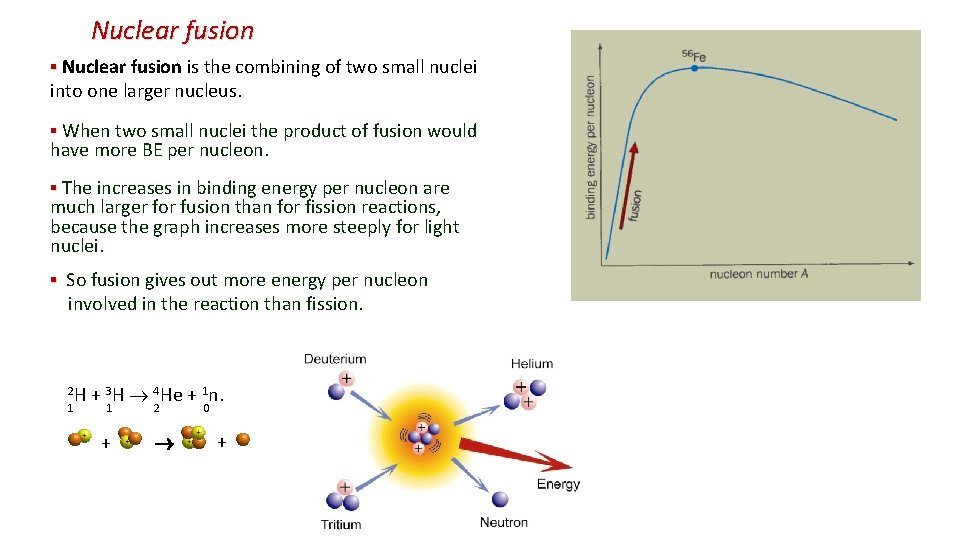Nuclear fusion ▪ Nuclear fusion is the combining of two small nuclei into one larger nucleus. ▪ When two small nuclei the product of fusion would have more BE per nucleon. ▪ The increases in binding energy per nucleon are much larger for fusion than for fission reactions, because the graph increases more steeply for light nuclei. ▪ So fusion gives out more energy per nucleon involved in the reaction than fission. 2 H + 3 H 4 He + 1 n. 1 1 2 0 + +Returning to the BE / A graph we see that iron (Fe) is the most stable of the nuclei. Nuclear fusion will occur with the elements below Fe because joining smaller nuclei forms bigger ones, which are higher on the graph (and thus more stable). Nuclear fission will occur with the elements above Fe because splitting bigger nuclei forms smaller ones, which are higher on the graph (and thus more stable).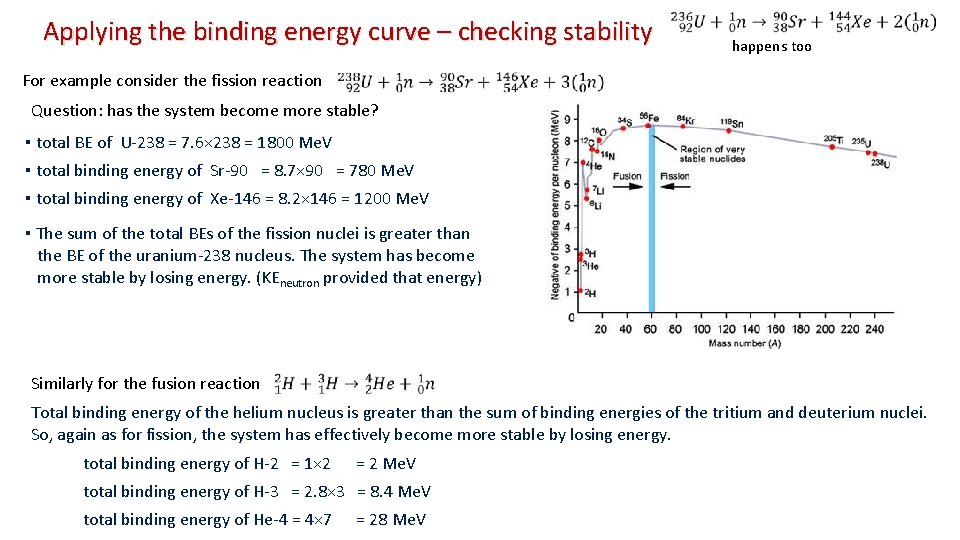Applying the binding energy curve – checking stability happens too For example consider the fission reaction Question: has the system become more stable? ▪ total BE of U-238 = 7. 6× 238 = 1800 Me. V ▪ total binding energy of Sr-90 = 8. 7× 90 = 780 Me. V ▪ total binding energy of Xe-146 = 8. 2× 146 = 1200 Me. V ▪ The sum of the total BEs of the fission nuclei is greater than the BE of the uranium-238 nucleus. The system has become more stable by losing energy. (KEneutron provided that energy) Similarly for the fusion reaction Total binding energy of the helium nucleus is greater than the sum of binding energies of the tritium and deuterium nuclei. So, again as for fission, the system has effectively become more stable by losing energy. total binding energy of H-2 = 1× 2 = 2 Me. V total binding energy of H-3 = 2. 8× 3 = 8. 4 Me. V total binding energy of He-4 = 4× 7 = 28 Me. V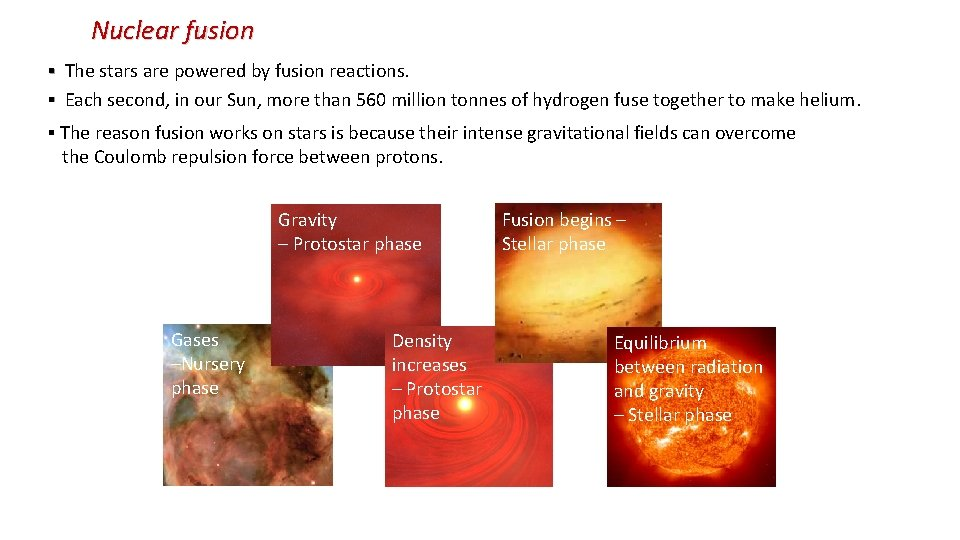Nuclear fusion ▪ The stars are powered by fusion reactions. ▪ Each second, in our Sun, more than 560 million tonnes of hydrogen fuse together to make helium. ▪ The reason fusion works on stars is because their intense gravitational fields can overcome the Coulomb repulsion force between protons. Gravity – Protostar phase Gases –Nursery phase Density increases – Protostar phase Fusion begins – Stellar phase Equilibrium between radiation and gravity – Stellar phaseNuclear fusion ▪ The gravitational force squishes hydrogen nuclei together overcoming the repulsive Coulomb force. ▪ The energy released by fusion prevents the gravitational collapse from continuing. ▪ Gravitation and radiation pressure reach equilibrium. ▪They balance each other.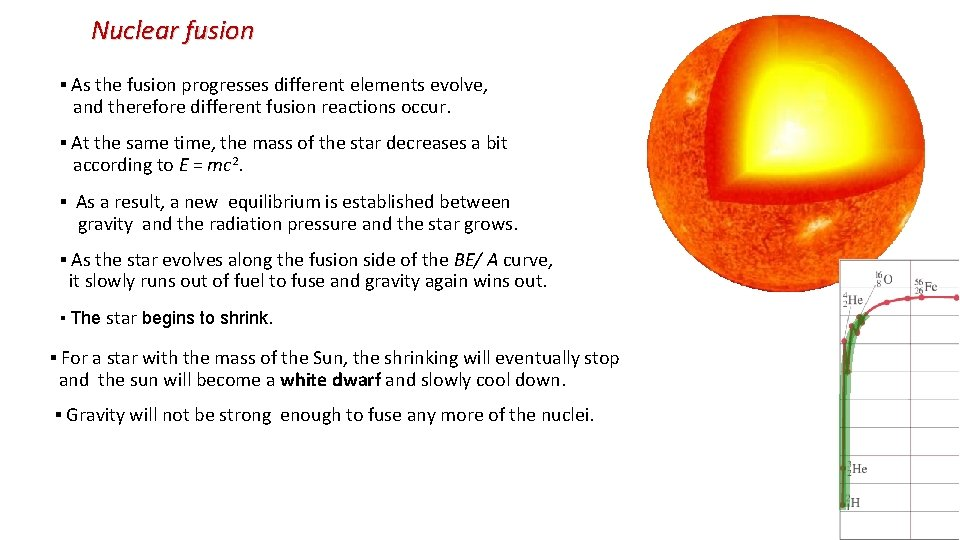Nuclear fusion ▪ As the fusion progresses different elements evolve, and therefore different fusion reactions occur. ▪ At the same time, the mass of the star decreases a bit according to E = mc 2. ▪ As a result, a new equilibrium is established between gravity and the radiation pressure and the star grows. ▪ As the star evolves along the fusion side of the BE/ A curve, it slowly runs out of fuel to fuse and gravity again wins out. ▪ The star begins to shrink. ▪ For a star with the mass of the Sun, the shrinking will eventually stop and the sun will become a white dwarf and slowly cool down. ▪ Gravity will not be strong enough to fuse any more of the nuclei.Nuclear fusion ▪ For a more massive star, there is enough gravity to fuse the elements all the way up to iron. ▪ But there can be no more fusion when the star is completely iron. Why? ▪ Since the radiation pressure now ceases, gravity is no longer balanced and the star collapses into a neutron star. ▪ This is called the iron catastrophe. ▪ Where do the elements above iron come from? ▪ For an even more massive star, there is enough gravity to overcome the neutron barrier to collapse. ▪ Collapse continues and nothing can stop it! ▪ The star becomes a black hole. ▪ A black hole is an extremely dense body whose gravitational force is so strong that even light cannot escape it! ▪Thus you cannot see it directly.Nuclear fusion ▪ scientists are keen to develop fusion as a source of power (fusion reactor). ▪ fusion has a number of advantages over fission: ▪ greater power output per kilogram, ▪ the raw materials are cheap and readily available, ▪ no radioactive elements are produced directly, ▪ irradiation by the neutrons leads to radioactivity in the reactor materials but these have relatively short half lives and only need to be stored safely for a short time. ▪ So why don't we use fusion in nuclear power stations? ▪ The JET (Joint European Torus) project was set up to carry out research into fusion power. ▪ It has yet to generate a self‑sustaining fusion reaction. ▪ The main problem is getting two nuclei close enough for long enough for them to fuse. ▪ To make the nuclei come close enough for the strong force to pull them together, they must be thrown together with very high velocity. For this to take place, the matter must either be heated to temperatures as high as the core of the sun (about 13 million Kelvin) ▪ At this temperature all matter exists as an ionised gas/plasma. ▪ Problem: containment. What can you use to hold something this hot? ▪ JET (and Princeton) uses magnetic fields in a doughnut shaped chamber called a torus to keep the plasma away from the container walls. ▪ Unfortunately generating high temperatures and strong magnetic fields uses up more energy than the fusion reaction produces!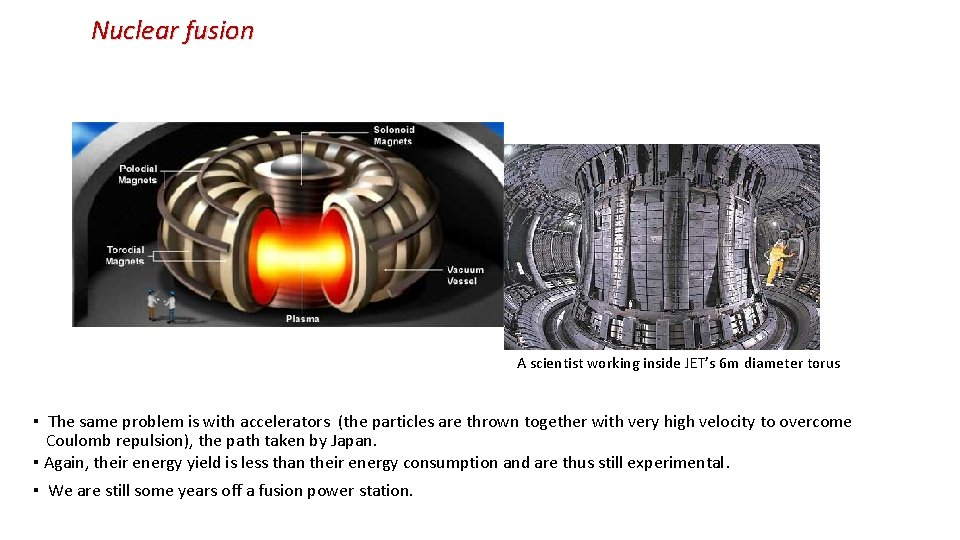Nuclear fusion A scientist working inside JET’s 6 m diameter torus ▪ The same problem is with accelerators (the particles are thrown together with very high velocity to overcome Coulomb repulsion), the path taken by Japan. ▪ Again, their energy yield is less than their energy consumption and are thus still experimental. ▪ We are still some years off a fusion power station.